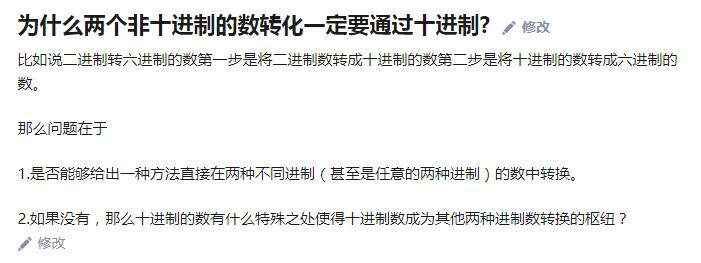1 × 8 + 1 × 4 + 0 × 2 + 0 × 1 = 12

2 + 6 = 11

3 + 4 = 10

2 * 2 = 4

$2^3=11$

1 × 11 + 1 × 4 + 0 × 2 + 0 × 1 = 11 + 4 = 15

ps: 上边的代码，没有做严格的测试，思想应该是对的，哈哈，发现问题可以和我交流。

## 总结一下

$$…a\times2^4+b\times2^3+c\times2^2+d\times2^1+e\times2^0=2020$$